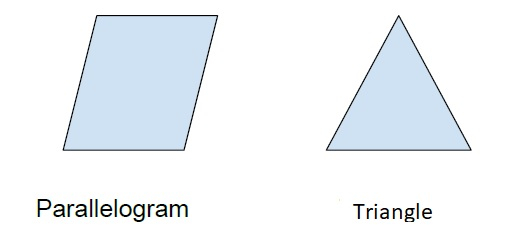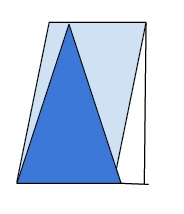# Area of a triangle inside a parallelogram in C++

The area of a figure is the extent of the figure in two-dimensional plane.

Triangle is a polygon with three sides.

Parallelogram is a quadrilateral with opposite sides equal and parallel.In this program, we have a parallelogram with its base and height and it inscribed a triangle the same base as the parallelogram. We need to calculate the area of the triangle using the given base and height.Area of triangle constructed take the base of a parallelogram and common height as the parallelogram is given by the formula = 0.5 * base * height

area = ½ * b * h

## Example

Live Demo

#include<iostream>
#include<math.h>
using namespace std;
int main(){
float b, h, Area;
b = 30.0;
h = 22.0;
Area = (0.5 * b * h);
cout<<"Area of triangle with base "<<b<<" and height "<<h<<" is "<<Area;
return 0;
}

## Output

Area of triangle with base 30 and height 22 is 330